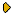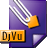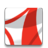Журнал Экспериментальной и Теоретической Физики
 НАЧАЛО | ПОИСК | ДЛЯ АВТОРОВ | ПОМОЩЬОбщая информация о журналеЗолотые страницыАдреса редакцииСодержание журналаСообщения редакцииПравила для авторовЗагрузить статьюПроверить статус статьиЖЭТФ, Том 123, Вып. 4, стр. 672 (Апрель 2003)
(Английский перевод - JETP, Vol. 96, No 4, p. 594, April 2003 доступен on-line на www.springer.com )

RADIATION FROM COSMIC CHIRAL STRING LOOPS

Поступила в редакцию: 13 Ноября 2002

PACS: 11.27.+d, 41.20.Jb, 04.30.-wDJVU (191.9K)PDF (433.8K)

The gravitational and electromagnetic radiation from chiral superconducting cosmic string loops is calculated. The formulas for energy, momentum, and angular momentum losses due to gravitational and electromagnetic radiation from chiral loops of an arbitrary configuration are derived. After summation over all modes, expressions for the corresponding radiation rates averaged over the loop oscillation period have the form of four-dimensional integrals. These formulas are reduced to sums over the kinks for loops composed of piecewise linear strings. For three examples of string loops, the total radiation rates are calculated numerically in dependence on the chiral current along the string. In the limit of a nearly maximum current, which corresponds to a stationary loop (vorton) configuration, we determine the upper bounds on the gravitational and electromagnetic radiation. We also estimate the oscillation damping time of a nearly stationary loop.

Сообщить о технических проблемах